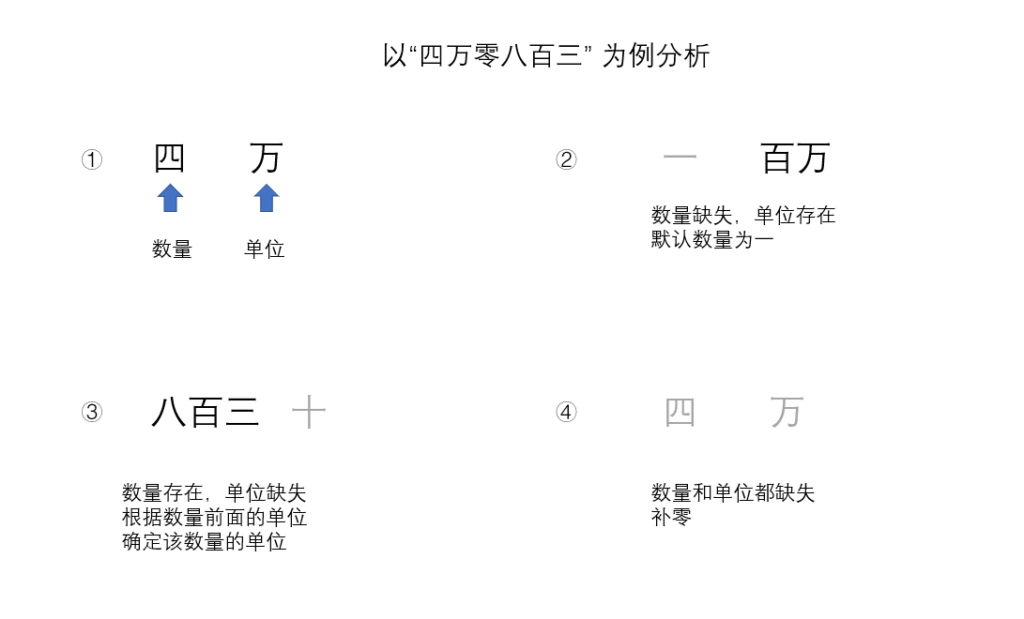# 汉字数字转换为阿拉伯数字```import re

def transfer(amount: str) -> str:
"""
amount: 提取到的价格的详细数字 例如：4百(万) 百(万) 千(万)
将amount标准化为：4百(万)->400(万)
"""
# 四百三十万
unit_list = ["兆", "千亿", "百亿", "十亿", "亿", "千万", "百万", "十万", "万", "千", "百", "十", "个"]
transfer_list = ["零", "一", "二", "三", "四", "五", "六", "七", "八", "九"]
real_amount = 0

if re.findall("^\d{1,}\$", amount):
return amount

amount_unit_index = -1
unit_list_index = -1
# 找到单位列表中的起始位置，即找到数字的最大单位
for index in range(0, len(amount)):
if amount[index:index + 2] in unit_list:
amount_unit_index = index
unit_list_index = unit_list.index(amount[index:index + 2])
break
elif amount[index] in unit_list:
amount_unit_index = index
unit_list_index = unit_list.index(amount[index])
break

# 数字字符串下标从头开始
amount_unit_index = 0
amount_result = ""
# 按照单位列表的顺序找，即按照单位从大到小的顺序提取数量
for index in range(unit_list_index, len(unit_list)):
# 如果当前单位在数字字符串中，那么从上次单位结束的位置开始查找和抽取
if unit_list[index] in amount[amount_unit_index::]:
result = re.findall("(.*?)" + unit_list[index], amount[amount_unit_index::])
if result != '':  # 抽到单位前面的数字了
print(result, unit_list[index], end="\t")
amount_result += result
else:  # 没抽到单位前面的数字但是单位确实存在，默认为一，比如我告诉你百万，默认是一百万
print('一', unit_list[index], end="\t")
amount_result += '一'
amount_unit_index += (len(unit_list[index]) + 1)
continue
# 如果单位不在数字字符串中，但是数量可能存在，例如八百三，这里的三就没有单位，但是可以依据三前面的单位提取
result = re.findall(unit_list[index - 1] + "(.)", amount[amount_unit_index - 1::])
if len(result) > 0:
print(result, unit_list[index], end="\t")
amount_result += result
amount_unit_index += 1
continue
# 上面两种情况都不存在 那就当前单位补零
print("零", unit_list[index], end="\t")
amount_result += "零"
# 将汉字替换为数字 四百->400
for index in range(0, len(transfer_list)):
amount_result = amount_result.replace(transfer_list[index], str(index))
return str(int(amount_result))

if __name__ == '__main__':
price_list = ["四十万零五百", "四千八百三", "零", "4331", "4百万", "4万", "百万", "百", "3万4", "3万四", "三万四", "三万4"]
result = []
for item in price_list:
result = transfer(item)
print('->', result)
print("")```

-> 4331
4 百万 零 十万 零 万 零 千 零 百 零 十 零 个 -> 4000000
4 万 零 千 零 百 零 十 零 个 -> 40000

3 万 [‘4’] 千 零 百 零 十 零 个 -> 34000
3 万 [‘四’] 千 零 百 零 十 零 个 -> 34000

Process finished with exit code 0